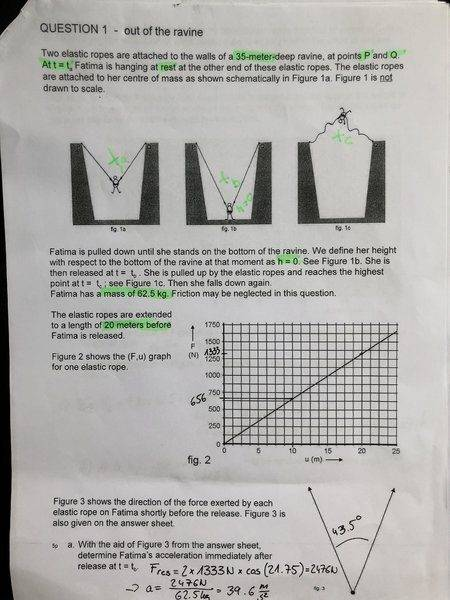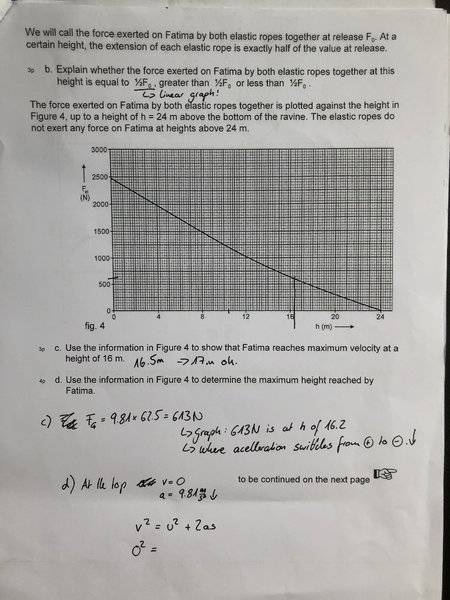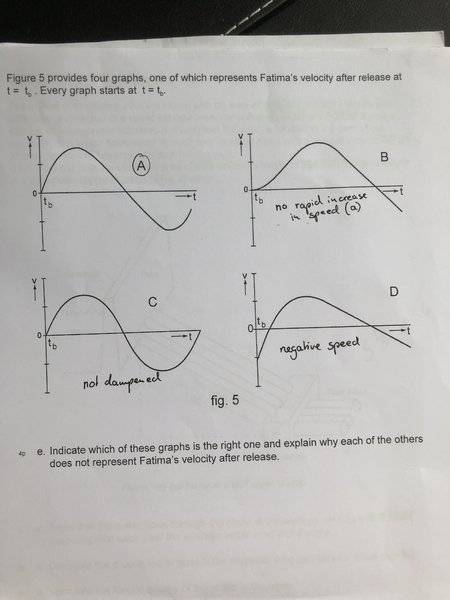# Two elastic ropes, Acceleration and Height Problem

## Homework Statement

As on the paper below:Suvat?

## The Attempt at a Solution

As on my paper

a) I think I got the acceleration correct by measuring the angle, halving the angle to get the force upwards by each rope. Or is that the wrong answer?

b) Should be half as it’s a linear graph right?

c) I guess I can read Fg off the graph. when Fg and Fel are equal the speed should be max.

d) No idea as to solve this as I’m missing too many variables for Suvat and I think suvat is only for constant a?

e) A seems right to me.

Been stuck on this 1 hour and would love your help! Thanks :)

#### Attachments

Last edited:

haruspex
Homework Helper
Gold Member
I think I got the acceleration correct by measuring the angle, halving the angle to get the force upwards by each rope.
I think you have done what was intended, but it is not really correct.
First, I'm not sure what it means by "the ropes are extended to a length of 20m". Clearly that is wrong since the ravine is 35m deep, so presumably it means extended by 20m. But is that 20m longer than the relaxed length or 20m longer then when Fatima was hanging at the equilibrium position?
Presumably it is the latter, but we do not know what angle the ropes made to the vertical in that position.
If they made angle α then the tension there, T0, satisfies 2T0cos(α)=mg. The extension by ΔL=20m gives a tension T0+kΔL. The initial acceleration on release would be 2(T0+kΔL)cos(β)/m-g=2kΔLcos(β)/m+g(sec(α)cos(β)-1).
Since α>β that is more than 2kΔLcos(β)/m.

Edit:
So maybe it means 20m longer than the relaxed length. This avoids the difficulty described above, but it also means the initial acceleration is much less than you calculated.

Last edited:
I think you have done what was intended, but it is not really correct.
First, I'm not sure what it means by "the ropes are extended to a length of 20m". Clearly that is wrong since the ravine is 35m deep, so presumably it means extended by 20m. But is that 20m longer than the relaxed length or 20m longer then when Fatima was hanging at the equilibrium position?
Presumably it is the latter, but we do not know what angle the ropes made to the vertical in that position.
If they made angle α then the tension there, T0, satisfies 2T0cos(α)=mg. The extension by ΔL=20m gives a tension T0+kΔL. The initial acceleration on release would be 2(T0+kΔL)cos(β)/m-g=2kΔLcos(β)/m+g(sec(α)cos(β)-1).
Since α>β that is more than 2kΔLcos(β)/m.

Ok thanks. I know what you mean. There is loads of vague / weird / incorrect wording in my opinion. Was my attempt at measuring correct though?

Thanks :)

haruspex
Homework Helper
Gold Member
b) Should be half as it’s a linear graph right?
Consider what I wrote in respect of a). How might that change the answer?
Note also that the second graph is not linear.

Consider what I wrote in respect of a). How might that change the answer?
Note also that the second graph is not linear.

Sorry but I don't know how I would calculate that. Sorry if it seems easy, but I have 0 knowledge coming into this.

haruspex
Homework Helper
Gold Member
Sorry but I don't know how I would calculate that. Sorry if it seems easy, but I have 0 knowledge coming into this.
It tells you that in the higher position the tensions have halved. What else has changed? Think about the geometry.

It tells you that in the higher position the tensions have halved. What else has changed? Think about the geometry.

The acceleration has halved too?

haruspex
Homework Helper
Gold Member
For c, what value are you using for g. With 10m/s2 I read the graph as saying maximum speed would be reached at 16.1-16.2m. With g as 9.8, even closer to 16m.

Forget it... i see that you revised it from 16.5-17 down to 16.2 already.

haruspex
Homework Helper
Gold Member
The acceleration has halved too?
That's not geometry.
Why was I going on about α and β in post #2?

haruspex
Homework Helper
Gold Member

Sorry I am still stuck. The more I look at it the more confused I am. What is Angle β?

Ahhhh regarding d)

Area under the Graph in fig.4 is Work. But the graph is not linear. Can I ignore that?

Then it would be 0.5 x 24m x 2476N = 29712Nm or J.

If I say at her highest point EGrav is max, I can say 29712J = m x g x h, giving me a height of 48.5m.

But the curve is not linear, so I'm wondering if I can use my above calculations....

haruspex
Homework Helper
Gold Member
What is Angle β?
43.5°/2.

haruspex